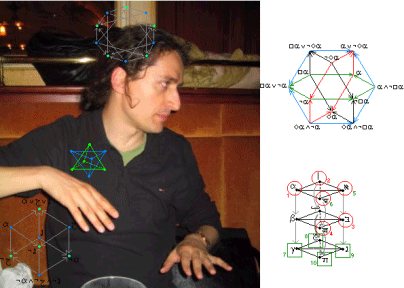The Geometry of Logical Oppositions

Alessio Moretti

Institute of Philosophy

University of Nice;Francen-opposition theory” (2004) generalises Aristotle’s opposition theory (exemplified by the “logical square”) by introducing the notion of “logical bi-simplex of dimension n”.

This theory, relevant to both quantification theory and modal logic (both are tied to the logical square) shows that there exists a field, between logic and geometry, where logical-geometrical n-dimensional solids (highly symmetrical structures whose edges are implication arrows), instead of being limited to the square (the poorest and ugliest of them), develop into infinite growing orders according some relatively simple but generally unknown principles.

This field is related to modal logic, in so much such n-dimensional structures can be decorated (as can the square) with arbitrary modalities via some suited decorating techniques (as the “modal n(m)-graphs”, or the “setting-method”, for instance).

The theory’s known applications, so far, concern mainly the study – from a new geometrical point of view –  of the known modal systems (normal or non-normal, abstract or applied), but also the study of the non-logical formalisms inspired by the logical square in psychology (cognitive science and psychoanalysis), linguistics (semiotics and pragmatics), philosophy (analytical as continental) and others.

In this tutorial we will introduce to the theory of n-opposition, showing (rapidly) its historical roots (Aristotle, Vasil’ev, Sesmat, Blanché), then concentrating both on its classical (recently discovered) tenets and on its actual (open) research issues. At the end of the tutorial, according to the time left, we will discuss of some of this theory’s possible applications.